May 26, 2020

In AMS, the filiamentous carbon or “graphite” derived from a sample is compressed into a small cavity in an aluminum “target” which acts as a cathode in the ion source. The surface of the graphite is sputtered with heated, ionized cesium and the ions produced are extracted and accelerated in the AMS system. After acceleration and removal of electrons, the emerging positive ions are magnetically separated by mass and the 12C and 13C ions are measured in Faraday Cups where a ratio of their currents is recorded. Simultaneously the 14C ions are recorded in a gas ionization (USAMS) or solid state (CFAMS) detector, so that ratios of 14C to 13C and 12C may be recorded. These are the raw signals that are ultimately converted to a radiocarbon age. From a contemporary sample, about 250 14C counts per second are collected. It is expected then, for a 5,700 year (1 half-life) or 11,400 year old (2 half-lives) sample that 125 or 63 counts per second would be obtained. Although one can simply measure older samples for longer times, there are practical limits to the minimum sample activity that can be measured.  At the present time, for a 1 milligram sample of graphite, this limiting age is about ten half-lives, or 60,000 years, if set only by the sample size. However, limiting ages or “backgrounds” are also determined by process blanks which correspond to the method used to extract the carbon from the sample. » NOSAMS General Statement of 14C Procedures

### Process Blanks

Process blanks are radiocarbon-free material that is prepared using the same methods as samples and standards. These blanks contain small but measurable amounts of 14C from contamination introduced during chemical preparation, collection or handling. Organic materials, which require the most processing, are limited to younger ages by their corresponding process blank. Due to counting and measurement errors for the blanks and samples, statistical errors are higher for very old samples. Thus, ages are limited by the age of the process blanks (more on that below) and by the statistical uncertainty of the 14C measurement.

### Blank corrected fraction modern

NOSAMS uses two blank correction methods, a mass-independent “large blank” and a mass balance correction that takes the proportion of blank relative to the sample size into account. The large blank correction assumes that the radiocarbon present in a process blank sample is mass-independent. For large samples, the blank corrected fraction modern (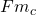) is computed from the expression: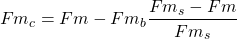Where (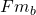), (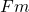) and (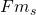) represent the 14C/12C ratios of the blank, the sample and the modern reference, respectively. For small samples, blank contribution as a fraction of sample mass becomes an even more important term. This correction is performed for all samples as follows: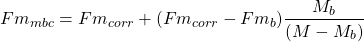Where (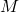) is sample mass, and (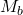) and () are the mass and Fm of the blank. Fraction Modern is a measurement of the deviation of the 14C/12C ratio of a sample from “Modern.” Modern is defined as 95% of the radiocarbon concentration (in AD 1950) of NBS Oxalic Acid I (SRM 4990B, OX-I) normalized to δ13CVPDB=-19 per mil (Olsson, 1970). AMS results are calculated using the internationally agreed upon definition of 0.95 times the specific activity of OX-I normalized to δ13CVPDB=-19 per mil. This is equivalent to an absolute (AD 1950) 14C/12C ratio of 1.176 ± 0.010 x 10-12 (Karlen, et. al., 1968); all results are normalized to -25 per mil using the δ13CVPDB of the sample (see below). The value used for this correction is specified in the report of final results.

### Fractionation Correction

In addition to loss through decay of radiocarbon, 14C is also affected by natural isotopic fractionation. Fractionation is the term used to describe the differential uptake of one isotope with respect to another. While the three carbon isotopes are chemically indistinguishable, lighter 12C atoms are preferentially taken up before the 13C atoms in biological pathways. Similarly, 13C atoms are taken up before 14C. The assumption is that the fractionation of 14C relative to 12C is twice that of 13C, reflecting the difference in mass. Fractionation must be corrected for in order to make use of radiocarbon measurements as a chronometric tool for all parts of the biosphere. In order to remove the effects of isotopic fractionation, the Fraction Modern is corrected to the value it would have if its original δ13C were -25 per mil (the δ13C value to which all radiocarbon measurements are normalized.) The fractionation correction is done using the 13/12 ratio measured by the AMS system. Using this measurement also corrects for any mass-dependent fractionation within the AMS system.

### Errors

The 14C atoms contained in a sample are directly counted using the AMS method of radiocarbon analysis. Accordingly, we calculate an internal statistical error using the total number of 14C counts (n) measured for each target (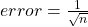). An external error is calculated from the reproducibility of multiple exposures for a given target. We measure the 14C /12C of a sample 10 separate times over the course of a run. The final reported error is the larger of the internal or external error, propagated with errors from the normalizing standards and blank subtraction. It should be noted that the reported error is an estimate of the precision (repeatability) of measurement for a single sample. Due to variability in sample homogeneity, sample collection, and sample processing, the variability of replicate samples (reproducibility) is generally greater than the reported error for a single sample. A total measurement error can be estimated by adding in quadrature the reported error with this extra variability, or added variance. At NOSAMS, added variance is determined by pooling differences of measurements of secondary standards from consensus values of those standards. For calendar year 2016, the estimated added variance for samples of the process type OC (Organic Carbon) or HY (Hydrolysis) is 2.6‰ for samples containing > 100 ug C. For other sample types, e.g. sample submitted as gas samples, dissolved inorganic or organic samples, samples with mass < 100 ug C, or reconnaissance gas ion source samples, an estimated added variance has not been determined. For water or dissolved inorganic carbon (DIC) samples, for which no internationally accepted secondary standards exist, we note that analyses of shipboard duplicates, collected on every cruise, demonstrate a pooled standard deviation of 3.0‰. This would indicate that the added variance for these samples is similar to other types of sample measured at NOSAMS. While added variance may give a better estimate of the total error, the best way to determine total experimental error is by replicate sample analyses. If you are working near the limits of AMS precision, or have questions regarding error estimates, please consult with us.

Radiocarbon age is calculated from the δ13C-corrected Fraction Modern according to the following formula: Age = -8033 ln (Fm) Reporting of ages and/or activities follows the convention outlined by Stuiver and Polach (1977) and Stuiver (1980). Ages are calculated using 5568 years as the half-life of radiocarbon and are reported without reservoir corrections or calibration to calendar years. For freeware programs, we suggest that you look at the following web site for a list of programs that will calibrate radiocarbon results to calendar years (including making reservoir corrections).[ Radiocarbon-Related Information Sources] The error in the age is given by 8033 times the relative error in the Fm . Therefore a 1% error in fraction-modern leads to an 80 year error in the age. Ages are rounded according to the convention of Stuiver & Polach, shown below.

### Rounding Convention

Age NearestErrorNearest
<10005<1005
1000-999910100-100010
10000-2000050>1000100
>20000100

### Limiting Ages

There are two situations that limit an age; the first is that the measured Fm is smaller than that of the corresponding process blank measured in the same suite of samples on the AMS. If this is the case, then the reported age will be quoted as an age greater than the age of the process blank. No age is reported greater than 60,000 years. The typical background age for organic combustions is 48,000 years and for inorganic carbon samples, 52,000 years. One other situation that limits the age (if not already limited by the background age) is the error of the AMS result. The reported age can be no older than the radiocarbon age of the fraction modern of the sample plus it’s error, as calculated by this formula: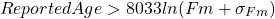### Age > Modern

Since Modern is defined as 95% of the 14C activity for AD 1950, as defined by the oxalic acid standard, sample activities can be substantially greater than Modern, and so the ages are reported as > Modern.

### Δ14C

We also report the Δ14C value as defined in Stuiver and Pollach (1977) as the relative difference between the absolute international standard (base year 1950) and sample activity corrected for age and δ13C. The Δ14C is age corrected to account for decay that took place between collection (or death) and the time of measurement so that two measurements of the same sample made years apart will produce the same calculated Δ14C result. Collection year must be specified in order for Δ14C results to be calculated.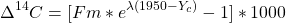Where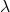is the inverse of the true mean-life of radiocarbon,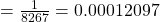and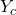is the year of collection.

### References

Karlen, I., Olsson, I.U., Kallburg, P. and Kilici, S., 1964. Absolute determination of the activity of two 14C dating standards. Arkiv Geofysik, 4:465-471.

Olsson, I.U., 1970. The use of Oxalic acid as a Standard. In I.U. Olsson, ed., Radiocarbon Variations and Absolute Chronology, Nobel Symposium, 12th Proc., John Wiley & Sons, New York, p. 17.

Stuiver, M. and Polach, H.A., 1977. Discussion: Reporting of 14C data. Radiocarbon, 19:355-363. (pdf)

Stuiver, M., 1980. Workshop on 14C data reporting. Radiocarbon, 22:964-966.

## General Statement of 14C Procedures at the National Ocean Sciences AMS Facility

May 25, 2020

This statement applies only to samples analyzed as solid graphite produced at the National Ocean Sciences AMS (NOSAMS) facility. It does not apply to samples analyzed on the gas ion source. Graphite is presently the most common target material.

An AMS radiocarbon measurement determines the ratio of 14C to 12C in an unknown sample relative to the known ratio in concurrently measured standards. The primary standard for 14C measurements is NBS Oxalic Acid I (NIST-SRM-4990). Every group of samples processed includes an appropriate blank, analyzed concurrently. Process blank materials include but are not limited to Carrara marble (IAEA C-1) and Icelandic Doublespar (Third International Radiocarbon Intercomparison F) for inorganic carbon and gas samples; acetanilide (CE Elantech) for organic carbon samples; 14C-free groundwater for dissolved inorganic carbon samples; and glycine (Sigma Aldrich) dissolved in DOC-free water for dissolved organic carbon samples.

Fraction modern (Fm) is the deviation of a sample’s radiocarbon content from that of the modern standard. Modern is defined as 95% of the radiocarbon concentration of NBS Oxalic Acid I normalized to δ13CVPDB = -19‰ (Olsson, 1970). An isotopic correction is made to normalize the sample result to a δ13CVPDB value of -25‰, assuming a quadratic mass fractionation dependency. This correction is made using 13C/12C ratios measured on the AMS system during radiocarbon analysis. These AMS 13C/12C ratios are not reported. Post-analysis stable isotopic corrections are neither necessary nor appropriate for reported 14C results. Reported δ13C values are measured on a split of sample CO2 using a dedicated stable isotope ratio mass spectrometer.

The 14C atoms contained in a sample are directly counted using the AMS method. Accordingly, we calculate an internal statistical error using the total number of 14C counts measured for each target (internal error=1/√n, where n is the number of 14C counts). An external error is calculated from the repeatability of multiple measurements of a given cathode over the course of a run (external error = standard deviation from the mean/√N, where N is the number of determinations). The final reported error is the larger of the internal or external error, propagated with errors from the normalizing standards and blank subtraction.

It should be noted that the reported error is an estimate of the precision (repeatability) of measurement for a single sample. Due to variability in sample homogeneity, sample collection, and sample processing, the variability of replicate samples (reproducibility) is generally greater than the reported error for a single sample. A total measurement error can be estimated by adding in quadrature the reported error with this extra variability, or added variance: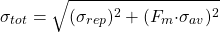where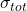is the total estimated error,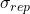is the reported error,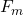is the measured Fraction Modern, and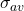is the appropriate added variance. At NOSAMS, added variance is determined by pooling differences of measurements of secondary standards from consensus values of those standards. For calendar year 2016, the estimated added variance for samples of the process type OC (Organic Carbon) or HY (Hydrolysis) is 2.6‰ for samples containing > 100 ug C. For other sample types, e.g. sample submitted as gas samples, dissolved inorganic or organic samples, samples with mass < 100 ug C, or reconnaissance gas ion source samples, an estimated added variance has not been determined. For water or dissolved inorganic carbon (DIC) samples, for which no internationally accepted secondary standards exist, we note that analyses of shipboard duplicates, collected on every cruise, demonstrate a pooled standard deviation of 3.0‰. This would indicate that the added variance for these samples is similar to other types of sample measured at NOSAMS. While added variance may give a better estimate of the total error, the best way to determine total experimental error is by replicate sample analyses. If you are working near the limits of AMS precision, or have questions regarding error estimates, please consult with us.

Radiocarbon ages are calculated using the Libby half-life of 5568 years according to the convention outlined by Stuiver and Polach (1977) and Stuiver (1980). We do not report ages with reservoir corrections applied or ages calibrated to calendar year. If a sample collection date is specified on the submittal form, the Δ14C activity normalized to 1950 is also reported, i.e. the activity or Δ14C of the sample is corrected to account for the decay between collection (or death) and the time of measurement.

When publishing results of samples analyzed at the NOSAMS facility, we ask that an accession number (i.e. the reported OS-#####) be listed with the result along with any subsequently made corrections. Published results should acknowledge support from NSF by including the NSF Cooperative Agreement number, OCE-1239667. We encourage you to email references to your publications to nosams_refs@whoi.edu for inclusion in our web-accessible database of NOSAMS research related  publications (http://nosamsresearch.whoi.edu/).

References

Olsson, I.U., 1970. The use of Oxalic acid as a Standard. In I.U. Olsson, ed., Radiocarbon Variations and Absolute Chronology, Nobel Symposium, 12th Proc., John Wiley & Sons, New York, p. 17.

Stuiver, M. and Polach, H.A., 1977. Discussion: Reporting of 14C data. Radiocarbon, 19:355-363.

Stuiver, M., 1980.Workshop on 14C data reporting. Radiocarbon, 22:964-966.